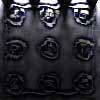#### You may also like### Fixing the Odds

You have two bags, four red balls and four white balls. You must put all the balls in the bags although you are allowed to have one bag empty. How should you distribute the balls between the two bags so as to make the probability of choosing a red ball as small as possible and what will the probability be in that case?### Scratch Cards

To win on a scratch card you have to uncover three numbers that add up to more than fifteen. What is the probability of winning a prize?### Escalator

At Holborn underground station there is a very long escalator. Two people are in a hurry and so climb the escalator as it is moving upwards, thus adding their speed to that of the moving steps. ... How many steps are there on the escalator?

# Guessing the Graph

##### Age 14 to 16Challenge Level

Can you find a quadratic curve that roughly fits Data Set A?
Where do you think the maximum point of the curve would be?
Consider how to turn the graph of $y=x^2$ upside down, move it up the axis, and 'squash' it to match the data.
This spreadsheet might be useful for trying out equations and seeing how closely they match the real data.